# Selina solutions for Concise Mathematics Class 9 ICSE chapter 17 - Circle [Latest edition]

#### Chapters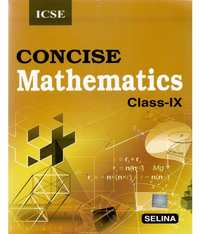## Chapter 17: Circle

Exercise 17 (A)Exercise 17 (B)Exercise 17 (C)Exercise 17 (D)
Exercise 17 (A) [Pages 210 - 211]

### Selina solutions for Concise Mathematics Class 9 ICSE Chapter 17 Circle Exercise 17 (A) [Pages 210 - 211]

Exercise 17 (A) | Q 1 | Page 210

A chord of length 6 cm is drawn in a circle of radius 5 cm.
Calculate its distance from the center of the circle.

Exercise 17 (A) | Q 2 | Page 210

A chord of length 8 cm is drawn at a distance of 3 cm from the center of the circle.
Calculate the radius of the circle.

Exercise 17 (A) | Q 3 | Page 210

The radius of a circle is 17.0 cm and the length of the perpendicular drawn from its center to a chord is 8.0 cm.
Calculate the length of the chord.

Exercise 17 (A) | Q 4 | Page 210

A chord of length 24 cm is at a distance of 5 cm from the center of the circle. Find the length of the chord of the same circle which is at a distance of 12 cm from the center.

Exercise 17 (A) | Q 5 | Page 210

In the following figure, AD is a straight line, OP ⊥ AD and O is the centre of both circles. If OA = 34cm, OB = 20 cm and OP = 16 cm;
find the length of AB.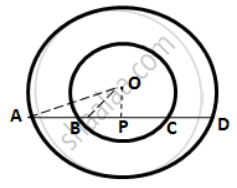Exercise 17 (A) | Q 6 | Page 210

In a circle of radius 17 cm, two parallel chords of lengths 30 cm and 16 cm are drawn. Find the distance between the chords,
if both the chords are:
(i) on the opposite sides of the centre;
(ii) on the same side of the centre.

Exercise 17 (A) | Q 7 | Page 210

Two parallel chords are drawn in a circle of diameter 30.0 cm. The length of one chord is 24.0 cm and the distance between the two chords is 21.0 cm;
find the length of another chord.

Exercise 17 (A) | Q 8 | Page 210

A chord CD of a circle whose center is O is bisected at P by a diameter AB. Given OA = OB = 15 cm and OP = 9 cm.
Calculate the lengths of: (i) CD ; (ii) AD ; (iii) CB.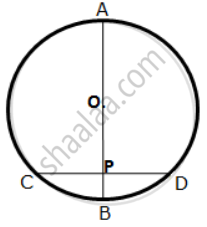Exercise 17 (A) | Q 9 | Page 211

The figure given below shows a circle with center O in which diameter AB bisects the chord CD at point E. If CE = ED = 8 cm and EB = 4 cm,
find the radius of the circle.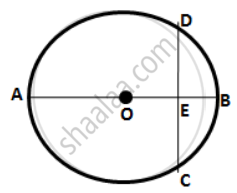Exercise 17 (A) | Q 10 | Page 211

In the given figure, O is the center of the circle. AB and CD are two chords of the circle. OM is perpendicular to AB and ON is perpendicular to CD. AB = 24 cm, OM = 5 cm, ON = 12 cm,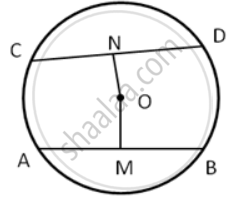Find the :
(i) the radius of the circle
(ii) length of chord CD.

Exercise 17 (B) [Page 217]

### Selina solutions for Concise Mathematics Class 9 ICSE Chapter 17 Circle Exercise 17 (B) [Page 217]

Exercise 17 (B) | Q 1 | Page 217

The figure shows two concentric circles and AD is a chord of a larger circle.
Prove that: AB = CD.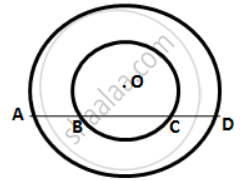Exercise 17 (B) | Q 2 | Page 217

A straight line is drawn cutting two equal circles and passing through the mid-point M of the line joining their centers O and O'. Prove that the chords AB and CD, which are intercepted by the two circles, are equal.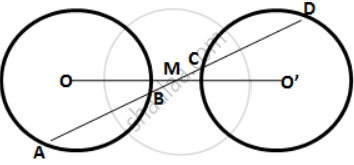Exercise 17 (B) | Q 3 | Page 217

M and N are the mid-points of two equal chords AB and CD respectively of a circle with center O.
Prove that: (i) ∠BMN = ∠DNM
(ii) ∠AMN = ∠CNM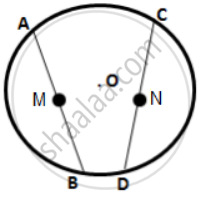Exercise 17 (B) | Q 4 | Page 217

In the following figure; P and Q are the points of intersection of two circles with centers O and O'. If straight lines APB and CQD are parallel to OO';
prove that: (i) OO' = 1/2AB ; (ii) AB = CD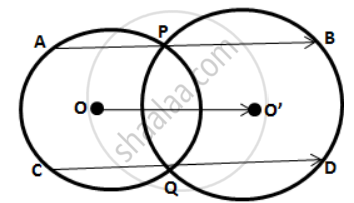Exercise 17 (B) | Q 5 | Page 217

Two equal chords AB and CD of a circle with center O, intersect each other at point P inside the circle.
Prove that: (i) AP = CP ; (ii) BP = DP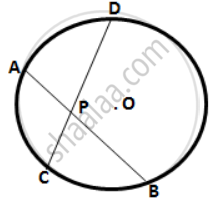Exercise 17 (B) | Q 6 | Page 217

In the following figure, OABC is a square. A circle is drawn with O as centre which meets OC at P and OA at Q.
Prove that:
( i ) ΔOPA ≅ ΔOQC
( ii ) ΔBPC ≅ ΔBQA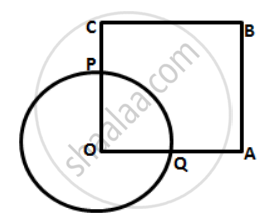Exercise 17 (B) | Q 7 | Page 217

The length of the common chord of two intersecting circles is 30 cm. If the diameters of these two circles are 50 cm and 34 cm, calculate the distance between their centers.

Exercise 17 (B) | Q 8 | Page 217

The line joining the midpoints of two chords of a circle passes through its center.
Prove that the chords are parallel.

Exercise 17 (B) | Q 9 | Page 217

In the following figure, the line ABCD is perpendicular to PQ; where P and Q are the centers of the circles.
Show that:
(i) AB = CD ;
(ii) AC = BD.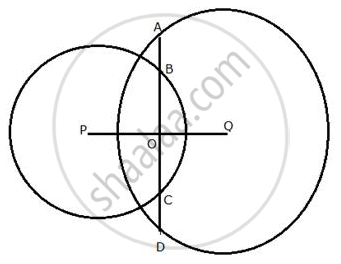Exercise 17 (B) | Q 10 | Page 217

AB and CD are two equal chords of a circle with center O which intersect each other at a right angle at point P.
If OM ⊥ AB and ON ⊥ CD;
show that OMPN is a square.

Exercise 17 (C) [Pages 220 - 221]

### Selina solutions for Concise Mathematics Class 9 ICSE Chapter 17 Circle Exercise 17 (C) [Pages 220 - 221]

Exercise 17 (C) | Q 1 | Page 220

In the given figure, an equilateral triangle ABC is inscribed in a circle with center O.
Find: (i) ∠BOC
(ii) ∠OBC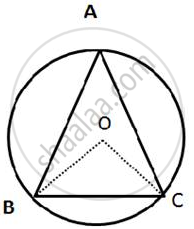Exercise 17 (C) | Q 2 | Page 220

In the given figure, a square is inscribed in a circle with center O.
Find:
(i) ∠BOC
(ii) ∠OCB
(iii) ∠COD
(iv) ∠BOD
Is BD a diameter of the circle?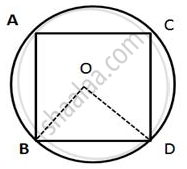Exercise 17 (C) | Q 3 | Page 220

In the given figure, AB is a side of regular pentagon and BC is a side of regular hexagon.
(i) ∠AOB
(ii) ∠BOC
(iii) ∠AOC
(iv) ∠OBA
(v) ∠OBC
(vi) ∠ABC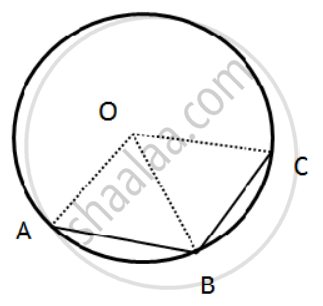Exercise 17 (C) | Q 4 | Page 220

In the given figure, arc AB and arc BC are equal in length. If ∠AOB = 48°, find:
(i) ∠BOC
(ii) ∠OBC
(iii) ∠AOC
(iv) ∠OAC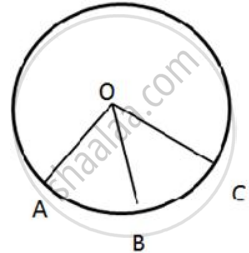Exercise 17 (C) | Q 5 | Page 220

In the given figure, the lengths of arcs AB and BC are in the ratio 3:2. If ∠AOB = 96°,
find: (i) ∠BOC (ii) ∠ABC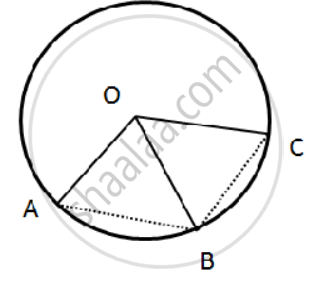Exercise 17 (C) | Q 6 | Page 220

In the given figure, AB = BC = DC and ∠AOB = 50°.
(i) ∠AOC
(ii) ∠AOD
(iii) ∠BOD
(iv) ∠OAC
(v) ∠ODA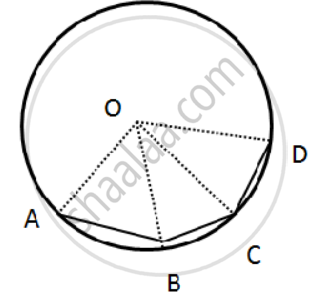Exercise 17 (C) | Q 7 | Page 221

In the given figure, AB is a side of a regular hexagon and AC is a side of a regular eight-sided polygon.
Find:
(i) ∠AOB
(ii) ∠AOC
(iii) ∠BOC
(iv) ∠OBC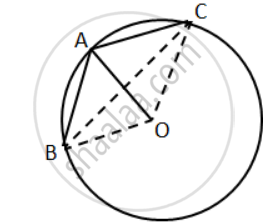Exercise 17 (C) | Q 8 | Page 221

In the given figure, O is the center of the circle and the length of arc AB is twice the length of arc BC. If ∠AOB = 100°,
find: (i) ∠BOC (ii) ∠OAC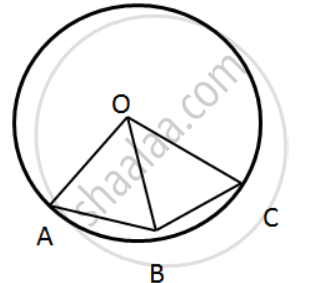Exercise 17 (D) [Page 221]

### Selina solutions for Concise Mathematics Class 9 ICSE Chapter 17 Circle Exercise 17 (D) [Page 221]

Exercise 17 (D) | Q 1 | Page 221

The radius of a circle is 13 cm and the length of one of its chords is 24 cm.
Find the distance of the chord from the center.

Exercise 17 (D) | Q 2 | Page 221

Prove that equal chords of congruent circles subtend equal angles at their center.

Exercise 17 (D) | Q 3 | Page 221

Draw two circles of different radii. How many points these circles can have in common? What is the maximum number of common points?

Exercise 17 (D) | Q 4 | Page 221

Suppose you are given a circle. Describe a method by which you can find the center of this circle.

Exercise 17 (D) | Q 5 | Page 221

Given two equal chords AB and CD of a circle with center O, intersecting each other at point P.
Prove that:
(i) AP = CP
(ii) BP = DP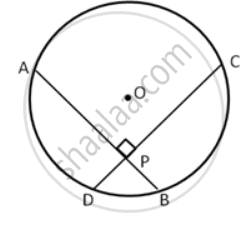Exercise 17 (D) | Q 6 | Page 221

In a circle of radius 10 cm, AB and CD are two parallel chords of lengths 16 cm and 12 cm respectively.
Calculate the distance between the chords, if they are on:
(i) the same side of the center.
(ii) the opposite sides of the center.

Exercise 17 (D) | Q 7 | Page 221

In the given figure, O is the center of the circle with radius 20 cm and OD is perpendicular to AB. If AB = 32 cm,
find the length of CD.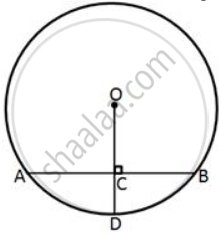Exercise 17 (D) | Q 8 | Page 221

In the given figure, AB and CD are two equal chords of a circle, with centre O. If P is the mid-point of chord AB, Q is the mid-point of chord CD and ∠POQ = 150°, find ∠APQ.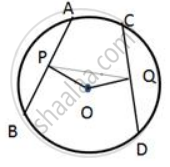Exercise 17 (D) | Q 9 | Page 221

In the given figure, AOC is the diameter of the circle, with centre O. If arc AXB is half of arc BYC, find ∠BOC.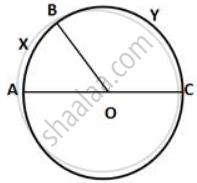Exercise 17 (D) | Q 10 | Page 221

The circumference of a circle, with center O, is divided into three arcs APB, BQC, and CRA such that:
"arc APB"/2 = "arc BQC"/3 = "arc CRA"/4

Find ∠BOC.

## Chapter 17: Circle

Exercise 17 (A)Exercise 17 (B)Exercise 17 (C)Exercise 17 (D)## Selina solutions for Concise Mathematics Class 9 ICSE chapter 17 - Circle

Selina solutions for Concise Mathematics Class 9 ICSE chapter 17 (Circle) include all questions with solution and detail explanation. This will clear students doubts about any question and improve application skills while preparing for board exams. The detailed, step-by-step solutions will help you understand the concepts better and clear your confusions, if any. Shaalaa.com has the CISCE Concise Mathematics Class 9 ICSE solutions in a manner that help students grasp basic concepts better and faster.

Further, we at Shaalaa.com provide such solutions so that students can prepare for written exams. Selina textbook solutions can be a core help for self-study and acts as a perfect self-help guidance for students.

Concepts covered in Concise Mathematics Class 9 ICSE chapter 17 Circle are Concept of Circle - Centre, Radius, Diameter, Arc, Sector, Chord, Segment, Semicircle, Circumference, Interior and Exterior, Concentric Circles, Arc, Segment, Sector, Chord Properties - a Straight Line Drawn from the Center of a Circle to Bisect a Chord Which is Not a Diameter is at Right Angles to the Chord, Concept of Circle - Centre, Radius, Diameter, Arc, Sector, Chord, Segment, Semicircle, Circumference, Interior and Exterior, Concentric Circles, Chord Properties - the Perpendicular to a Chord from the Center Bisects the Chord (Without Proof), Theorem: Equal chords of a circle are equidistant from the centre., Converse: The chords of a circle which are equidistant from the centre are equal., Chord Properties - There is One and Only One Circle that Passes Through Three Given Points Not in a Straight Line, Arc and Chord Properties - If Two Arcs Subtend Equal Angles at the Center, They Are Equal, and Its Converse, Concept of Circle - Centre, Radius, Diameter, Arc, Sector, Chord, Segment, Semicircle, Circumference, Interior and Exterior, Concentric Circles.

Using Selina Class 9 solutions Circle exercise by students are an easy way to prepare for the exams, as they involve solutions arranged chapter-wise also page wise. The questions involved in Selina Solutions are important questions that can be asked in the final exam. Maximum students of CISCE Class 9 prefer Selina Textbook Solutions to score more in exam.

Get the free view of chapter 17 Circle Class 9 extra questions for Concise Mathematics Class 9 ICSE and can use Shaalaa.com to keep it handy for your exam preparation